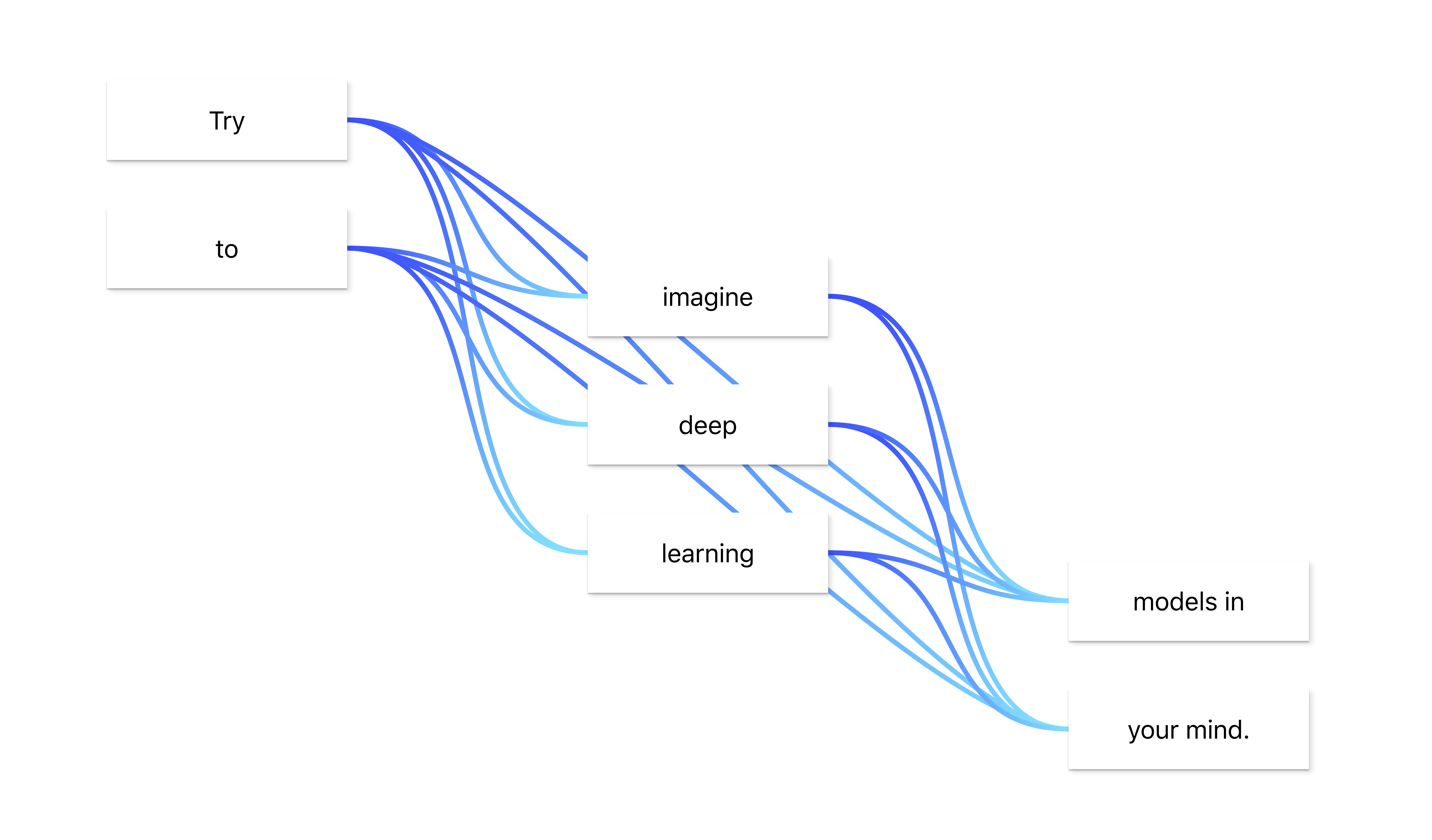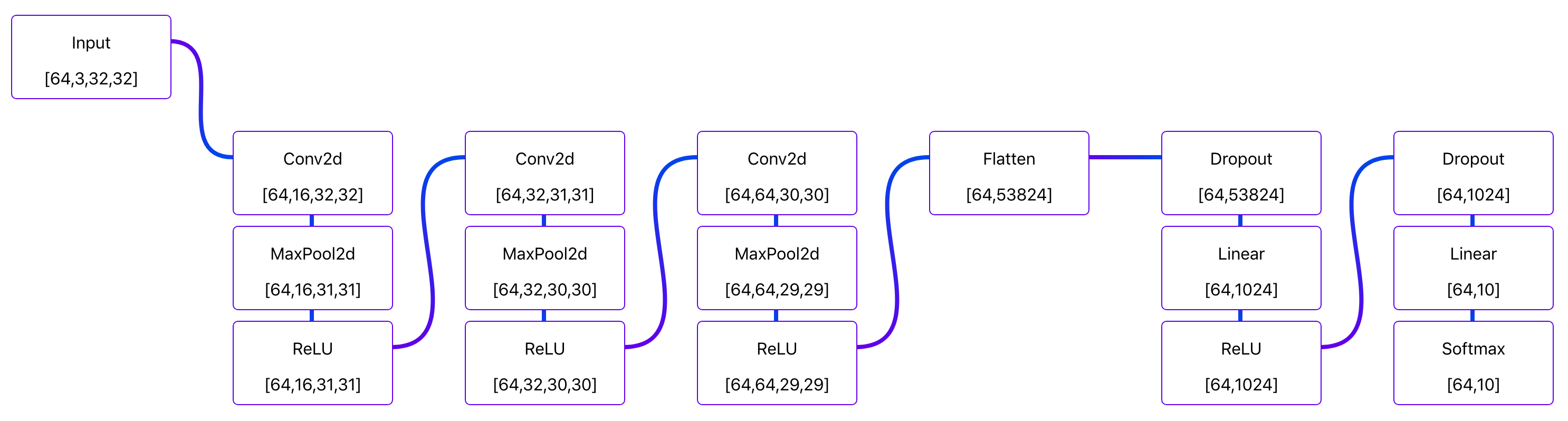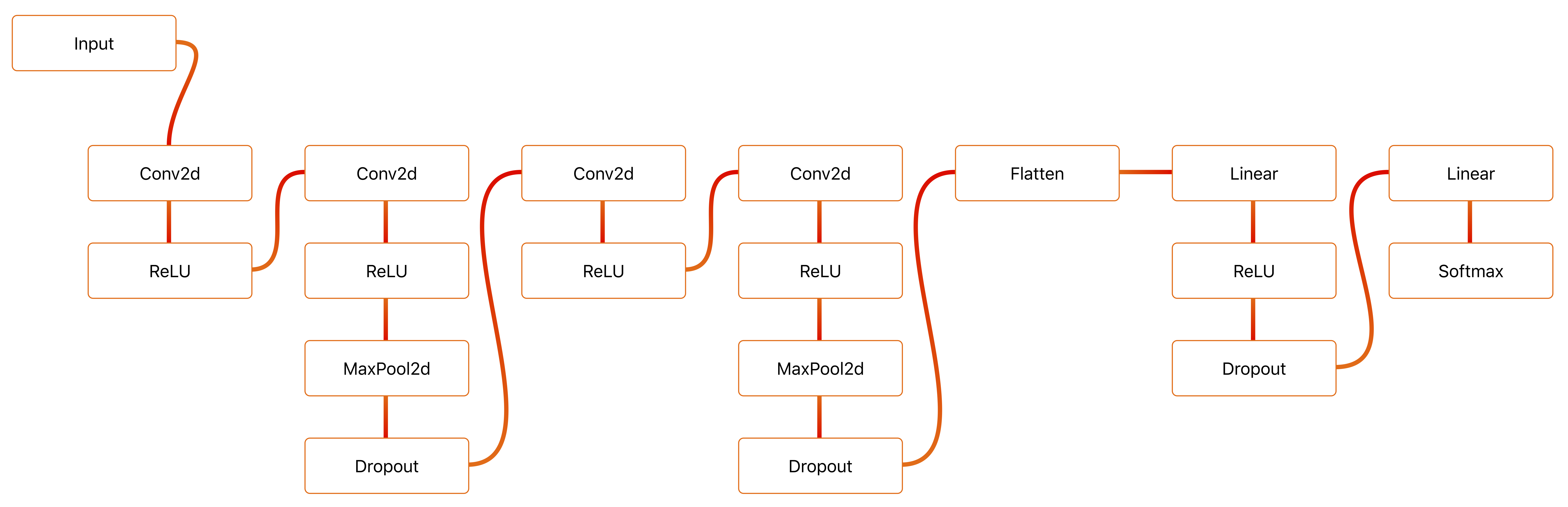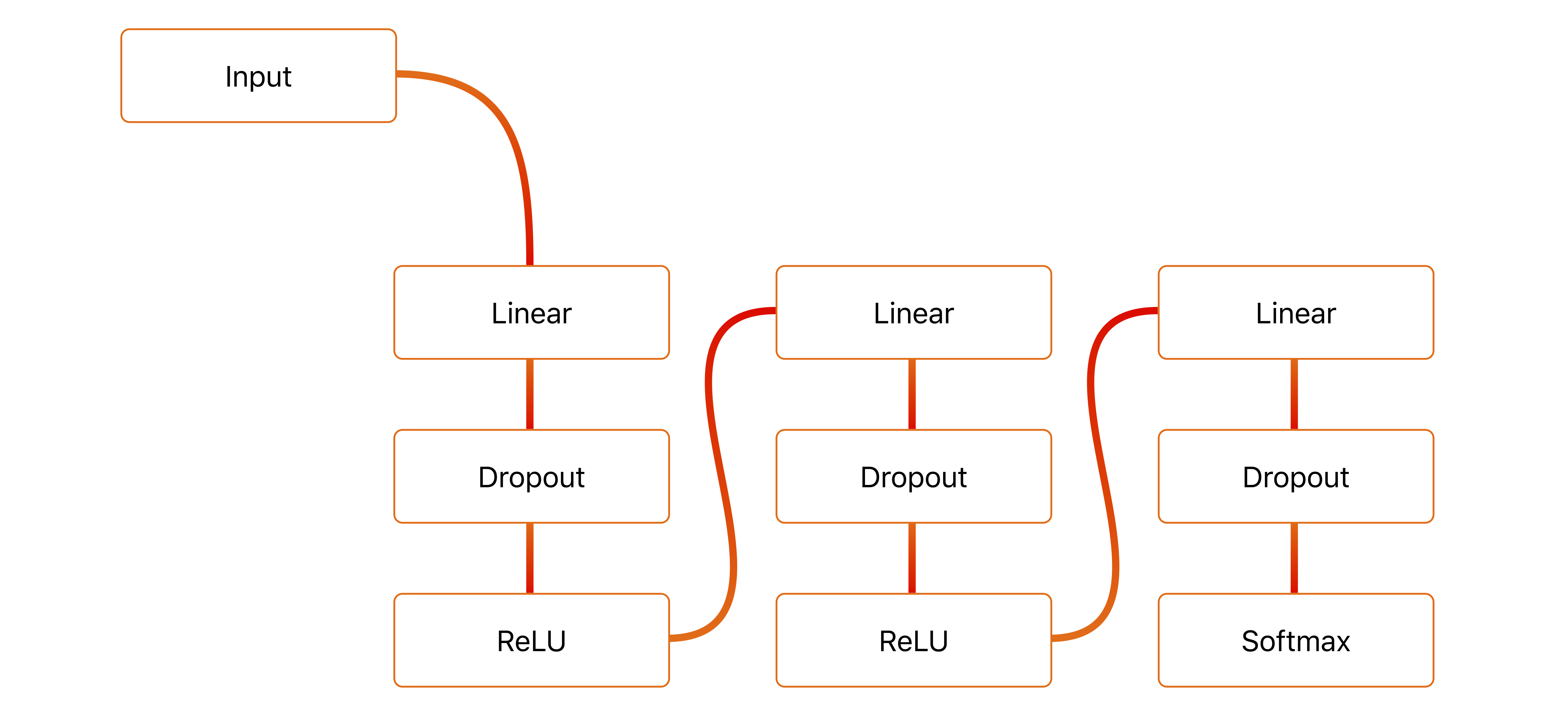# Flowpoints

Create deep learning models without all the typing and dimension mismatches! Follow this link to play around with this on your own :)

This npm package is a spin-off of this project, which this project is now utilizing.

Here's a colab using this PyTorch model.And here's similar colab using this TensorFlow model## Overview

This project is used to host a website in which users can quickly create drafts for deep learning models and have the equivalent plug-and-play code output immediately.

The code output to the user is written in python and utilizes PyTorch and TensorFlow.

## User guide

Or maybe just play around with it yourself?

### How I use these diagrams

1. Start by adding an input block and edit it's parameters to get the correct number of dimensions and features.
2. Add a bunch of blocks, organize them a little bit and add all connections.
3. Start from the top and move downstream, editing block parameters to ensure their outputs match the desired dimensions.
4. Add names to all blocks (if needed).
5. Quickly review code.
6. Copy code, plug it into existing pipeline, lean back, and watch the magic.

### Building new models

In the lower left corner of the website there are five buttons. These can be used to add new flowpoints (blocks) to the model, copy code to clipboard, generate a link to the current model, hide/show sidebar, and display a dialog to help users get started.

To build a new model start by clicking the blue + button. This will add a Flowpoint.

Click the + button a few more times to add more points. These will automatically connect if you don't click anywhere else on the screen.

To connect one Flowpoint to another, start by clicking on the first point. Then hold shift while clicking the second point. The points should now be connected. To disconnect the two simply repeat this process.

#### Changing the parameters of your model

Each flowpoint represents one operation in your model. To edit a Flowpoint's function and parameters, click the "Flowpoint"-tab in the sidebar (if the sidebar is not showing click the menu-button in the bottom left of your screen).

The top field in the sidebar allow you to change the operation of the selected flowpoint. Simply click it to display a list of all available operations.

In the second field you can enter a name for your flowpoint. If you don't type a name your flowpoint will be named p_1, p_2, p_3, etc.. in the output python code.

In the parameters area all the available parameters of the flowpoint can be viewed, and most of them can be changed. The "in_features" field will automatically be set to a valid size.

### Changing appearance

In the "Settings"-tab you can change the appearance of your diagrams. Theme and variant changes the look of your flowpoints, while the switches change the background and what information your flowpoints should display.

### Extracting the model code

If you click the "Code"-tab you'll see all the code for your model. The easiest way to copy this code to your clipboard is by clicking the "copy"-button, just beneath the + button.

## Example output

The following code was generated for this model.In TensorFlow:

```'''
Created using flowpoints.io

https://mariusbrataas.github.io/flowpoints_ml/?p=A4xw9sjChG9B

'''

# Importing TensorFlow tools
import tensorflow as tf

# Model

# Initializing inputs
in_data = tf.keras.layers.Input((1,10,), name='in_data')

# Initializing layers
fc1 = tf.keras.layers.Dense(
name               = "fc1",
units              = 96,
use_bias           = True,
kernel_initializer = "glorot_uniform",
bias_initializer   = "zeros",
)(in_data)
do1 = tf.keras.layers.Dropout(
name = "do1",
rate = 1,
)(fc1)
act1 = tf.keras.layers.ReLU(
name           = "act1",
negative_slope = 0,
threshold      = 0,
)(do1)
fc2 = tf.keras.layers.Dense(
name               = "fc2",
units              = 96,
use_bias           = True,
kernel_initializer = "glorot_uniform",
bias_initializer   = "zeros",
)(act1)
do2 = tf.keras.layers.Dropout(
name = "do2",
rate = 1,
)(fc2)
act2 = tf.keras.layers.ReLU(
name           = "act2",
negative_slope = 0,
threshold      = 0,
)(do2)
fc3 = tf.keras.layers.Dense(
name               = "fc3",
units              = 5,
use_bias           = True,
kernel_initializer = "glorot_uniform",
bias_initializer   = "zeros",
)(act2)
do3 = tf.keras.layers.Dropout(
name = "do3",
rate = 1,
)(fc3)
act3 = tf.keras.layers.Softmax(
name = "act3",
axis = -1,
)(do3)

# Creating model
_model = tf.keras.models.Model(
inputs  = [in_data],
outputs = [act3],
name    = 'flowpoints.io/?p=A4xw9sjChG9B'
)

# Compiling model
_model.compile(
optimizer = optimizer,
loss      = loss,
metrics   = metrics
)

# Returning model
return _model
```

Or similarly, in PyTorch:

```'''
Created using flowpoints.io

https://mariusbrataas.github.io/flowpoints_ml/?p=A4xw9sjChG9B

'''

# Importing PyTorch tools
import torch
from torch import nn, optim, cuda

# Importing other libraries
import numpy as np
import matplotlib.pyplot as plt
import time

# Model
class NeuralNet(nn.Module):

def __init__(self, optimizer=optim.SGD, alpha=0.01, criterion=nn.CrossEntropyLoss(), use_cuda=None):

# Basics
super(NeuralNet, self).__init__()

# Settings
self.optim_type = optimizer
self.optimizer  = None
self.alpha      = alpha
self.criterion  = criterion

# Use CUDA?
self.use_cuda = use_cuda if (use_cuda != None and cuda.is_available()) else cuda.is_available()

# Current loss and loss history
self.train_loss      = 0
self.valid_loss      = 0
self.train_loss_hist = []
self.valid_loss_hist = []

# Initializing all layers
self.fc1 = nn.Linear(
in_features  = 10,
out_features = 96,
bias         = False,
)
self.do1 = nn.Dropout(
p       = 0.25,
inplace = False,
)
self.act1 = nn.ReLU(
inplace = False,
)
self.fc2 = nn.Linear(
in_features  = 96,
out_features = 96,
bias         = False,
)
self.do2 = nn.Dropout(
p       = 0.25,
inplace = False,
)
self.act2 = nn.ReLU(
inplace = False,
)
self.fc3 = nn.Linear(
in_features  = 96,
out_features = 5,
bias         = False,
)
self.do3 = nn.Dropout(
p       = 0.25,
inplace = False,
)
self.act3 = nn.Softmax()

# Running startup routines
self.startup_routines()

def startup_routines(self):
self.optimizer = self.optim_type(self.parameters(), lr=self.alpha)
if self.use_cuda:
self.cuda()

def predict(self, in_data):

# Use CUDA?
if self.use_cuda:
in_data = in_data.cuda()

# Running inference
return self.forward(in_data)

def forward(self, in_data):
in_data = self.fc1(in_data)     # Linear
in_data = self.do1(in_data)     # Dropout
in_data = self.act1(in_data)    # ReLU
in_data = self.fc2(in_data)     # Linear
in_data = self.do2(in_data)     # Dropout
in_data = self.act2(in_data)    # ReLU
in_data = self.fc3(in_data)     # Linear
in_data = self.do3(in_data)     # Dropout
self.state = self.act3(in_data) # Softmax
return self.state

# Preparations for fit step
self.train_loss = 0 # Resetting training loss

# Looping through data

# Use CUDA?
if self.use_cuda:
in_data = in_data.cuda()
act3_target = act3_target.cuda()

# Forward pass
self.forward(in_data)

# Calculating loss
loss = self.criterion(self.state, act3_target)
self.train_loss += loss.item() # Adding to epoch loss

# Backward pass and optimization
loss.backward()                      # Backward pass
self.optimizer.step()                # Optimizing weights

# Preparations for validation step
self.valid_loss = 0 # Resetting validation loss

# Looping through data

# Use CUDA?
if self.use_cuda:
in_data = in_data.cuda()
act3_target = act3_target.cuda()

# Forward pass
self.forward(in_data)

# Calculating loss
loss = self.criterion(self.state, act3_target)
self.valid_loss += loss.item() # Adding to epoch loss

# Helpers
best_validation = 1e5

# Possibly prepping progress message
if show_progress:
epoch_l = max(len(str(epochs)), 2)
print('Training model...')
print('%sEpoch   Training loss   Validation loss   Duration   Time remaining' % ''.rjust(2 * epoch_l - 4, ' '))
t = time.time()

# Looping through epochs
for epoch in range(epochs):
if validation_loader != None:  # Perform validation?

# Possibly printing progress
if show_progress:
eta_s = (time.time() - t) * (epochs - epoch)
eta = '%sm %ss' % (round(eta_s / 60), 60 - round(eta_s % 60))
print('%s/%s' % (str(epoch + 1).rjust(epoch_l, ' '), str(epochs).ljust(epoch_l, ' ')),
'| %s' % str(round(self.train_loss_hist[-1], 8)).ljust(13, ' '),
'| %s' % str(round(self.valid_loss_hist[-1], 8)).ljust(15, ' '),
'| %ss' % str(round(time.time() - t, 3)).rjust(7, ' '),
'| %s' % eta.ljust(14, ' '))
t = time.time()

# Possibly saving model
if save_best:
if self.valid_loss_hist[-1] < best_validation:
self.save('best_validation')
best_validation = self.valid_loss_hist[-1]

# Switching to eval
self.eval()

def plot_hist(self):

plt.plot(self.train_loss_hist, color='blue', label='Training loss')
plt.plot(self.valid_loss_hist, color='springgreen', label='Validation loss')

# Axis labels
plt.xlabel('Epoch')
plt.ylabel('Loss')
plt.legend(loc='upper right')

# Displaying plot
plt.show()

def save(self, name='model.pth'):
if not '.pth' in name: name += '.pth'
torch.save({
'fc1': self.fc1,
'do1': self.do1,
'act1': self.act1,
'fc2': self.fc2,
'do2': self.do2,
'act2': self.act2,
'fc3': self.fc3,
'do3': self.do3,
'act3': self.act3,
'train_loss':      self.train_loss,
'valid_loss':      self.valid_loss,
'train_loss_hist': self.train_loss_hist,
'valid_loss_hist': self.valid_loss_hist,
'optim_type':      self.optim_type,
'alpha':           self.alpha,
'criterion':       self.criterion,
'use_cuda':        self.use_cuda
}, name)

@staticmethod
if not '.pth' in name: name += '.pth'
model = NeuralNet(
optimizer = checkpoint['optim_type'],
alpha     = checkpoint['alpha'],
criterion = checkpoint['criterion'],
use_cuda  = checkpoint['use_cuda']
)
model.fc1 = checkpoint['fc1']
model.do1 = checkpoint['do1']
model.act1 = checkpoint['act1']
model.fc2 = checkpoint['fc2']
model.do2 = checkpoint['do2']
model.act2 = checkpoint['act2']
model.fc3 = checkpoint['fc3']
model.do3 = checkpoint['do3']
model.act3 = checkpoint['act3']
model.train_loss      = checkpoint['train_loss']
model.valid_loss      = checkpoint['valid_loss']
model.train_loss_hist = checkpoint['train_loss_hist']
model.valid_loss_hist = checkpoint['valid_loss_hist']
model.startup_routines()
return model
```

## Contributing to this project

I'd love some help maintaining this project or adding more and better functionality!

The code is maintained in the master branch, while the website is hosted from the gh-pages branch.

As of right now the code is not properly commented, but I'll do that pretty soon.

Some ideas I'd like to implement:

• Pre-processing. Maybe something utilizing the torch data loader, with support for multiple workers and use of transforms.
• Better support for mobile. Not so great on my phone, kinda ok on my iPad.
• Python load model by link?? Maybe not possible, but would be awesome!

Also, this project is structured in a somewhat okay manner, but I'm not entirely happy with it, and might change it soon.

``````flowpoints_ml
├── .gitattributes
├── .gitignore
├── package-lock.json
├── package.json
│
├── public
│   ├── favicon.ico
│   ├── index.html
│   └── manifest.json
│
└── src
├── App.css
├── App.js
├── Cryptographer.js
├── DataBaseHandler.js
├── HelpDialog.js
├── Helpers.js
├── index.css
├── index.js
├── LibraryParser.js
├── MainButtons.js
├── MainLibrary.js
├── serviceWorker.js
│
├── libraries
│   ├── base_library.js
│   ├── pytorch_autoparams.js
│   ├── pytorch_library.js
│   └── tensorflow_library.js
│
├── parser
│   ├── FlowOrder.js
│   ├── Parser.js
│   ├── PyTorchParser.js
│   └── TensorflowParser.js
│
└── sidebar
├── CodeTab.js
├── FlowpointTab.js
├── FrontHelpers.css
├── FrontHelpers.js
├── MiscTab.js
├── Sidebar.css
├── Sidebar.js
├── SidebarContents.css
├── SidebarContents.js
``````

## Dependencies

Current dependencies in this project are:

MIT

Get A Weekly Email With Trending Projects For These Topics
No Spam. Unsubscribe easily at any time.
javascript (67,533
python (50,132
react (5,065
deep-learning (3,698
machine-learning (3,388
pytorch (2,153
tensorflow (2,078
js (492
flowchart (41